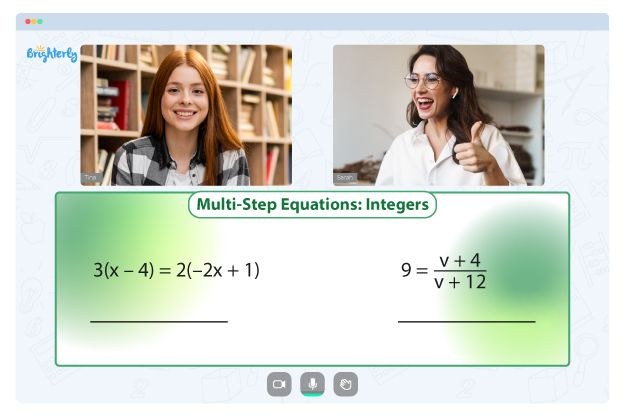# Factoring Trinomials Worksheets

Students frequently struggle when they encounter trinomials for the first time. Exposing kids to valuable educational resources will help them understand this math concept faster. The factoring perfect square trinomials worksheet is a practical resource to help your kids master the idea of trinomials. A factoring trinomials of the form ax2 bx c worksheet comprises numerous tasks your kid will solve and enhance their understanding of the topic.

## The concept of trinomials

Monomials, polynomials, binomials, and trinomials are categorized as algebraic expressions. Trinomials are always represented in quadratic form as ax² + bx + c. The most popular method is the “ac” format in factoring trinomials.

Remember that the “ac” format is simply one strategy and is not applicable in some instances. The formula states that the lead coefficient “a” should be multiplied by the constant “c.” If we look at the equation 2x² + 10x – 6, we can solve it by multiplying the a value (which is 2) by the c value (-6), resulting in the new equation x² + 10x – 12.

Trinomials have three parts (“tri” in the word implies three): the pure constant, squared variable, and single variable. The trinomial expression uses separators ×, +, –, and ÷.

## Content of a factoring perfect square trinomials worksheet

The prime factors and factoring trinomial worksheets cover concepts like prime factors, LCMs (Lowest Common Multiples), and GCFs/HCF (Greatest/Highest Common Factors). Irrespective of your kid’s age, the worksheets comprise questions of varying difficulty levels.

The following are topics your kids will encounter in the factoring trinomials worksheets.

### Factoring trinomials worksheets for Grade 4

• Calculating the factors of numbers from 4 to 50
• Calculating the factors of numbers from 4 to 100
• Multiples of whole numbers
• Prime factors and trees
• Divisibility rules

Math for Kids

Is Your Child Struggling With Math?
1:1 Online Math Tutoring### Factoring trinomials worksheets for fifth grade

• Factoring digits to prime factors
• Prime Factor trees
• Focus on the rules of divisibility
• The GCF of two digits from 1 to 100 or 1 to 50
• The LCM of two digits from 2 to 30
• The LCM and GCF arithmetic word problems

### Factoring worksheet for sixth graders

• The GCF of two digits up to 100
• The GCF of three digits up to 100
• The LCM of two digits up to 50
• The LCM of three digits up to 30.
• Factor digits (up to 500) to prime factors

For grade seven kids, the factoring trinomials worksheet answers comprise problems on concepts like factoring quadratic trinomials and dividing the middle term.

## Free factoring trinomials of the form ax2+bx+c worksheet### Factoring Trinomials Worksheets PDF

Factoring Trinomials Worksheet### Factoring Trinomials Worksheets PDF

Factoring Trinomials Of The Form Ax2+Bx+C Worksheet### Factoring Trinomials Worksheets PDF

Factoring Trinomials A 1 Worksheet Answers### Factoring Trinomials Worksheets PDF

Worksheets focusing on factoring trinomials can significantly improve pupils’ mastery of this skill. The worksheets are essential to help kids learn and master how to solve problems with trinomials. As a subfield of polynomials, trinomials are so vital that no one should ignore them.

To help your kid learn effectively and be top in their class, you must unleash valuable resources like the worksheet factoring trinomials from Brighterly. Brighterly’s factoring trinomial worksheets enhance learning through excitement and pleasure. Relying on textbooks can not be a sufficient means of understanding the trinomials.

## Printable factoring trinomials a 1 worksheet answers

You can get the printable factoring trinomials a 1 worksheet answers from the Brighterly’s website as an educator or parent. You can download the PDF version and print it in a paper format to allow easy access. Then, teach your kids to study independently with the paper worksheet.

### More Factoring Worksheets

Struggling with Equations?• Is your child having trouble mastering equations?
• An online tutor could provide the necessary support.

Does your child need extra help with understanding the concept of equations? Start studying with an online tutor.